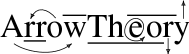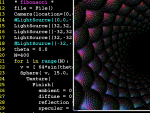# PyPov: python for povray

Here is a relatively simple framework for making povray files from your favourite programming language, python. It's good for creating structured/mathematical scenes and animations.

### Tutorial

1) Some code snippets.
`Sphere( (0,0,0), 1, color=(1,1,1) )`
`Union( Sphere(v1,r), Sphere(v2,r) )`
2) To change/set named args:
```torus = Torus( 4, 1 )
torus.rotate = 90*x # x is actually a Vector instance ```
And unnamed args via their index (untested):
`torus = 8 # bigger now `
3) Use the File object to output these to a pov file.
```file = File( "out.pov" )
torus.write(file)
torus.rotate = 90 * x
torus.write(file) # another torus ```
Here is a complete example for a chain of spheres:
```def test04():
cam = Camera(
location=(0,0,-10),
look_at=(100.0,0.0,0.0),
angle=30 )
light = LightSource((300,300,-1000),(1,1,1))
spheres = [ Sphere( (2*i,0,0), 1, Pigment(color=(1,1,1)) )
for i in range(400) ]
file = File( "spheres.pov", cam, light, *spheres )
```
Here is the pond:
```  water = Texture(
Finish(
ambient = 0.2,
diffuse = 0.3,
reflection = 0.8,
specular = 0.3
), Pigment(color=(0.4,0.4,1)))
def field(x,y):
x-=0.5; y-=0.5;
return (1.0+sin(6.0*sqrt(x*x+y*y)*2*pi))/2.0
FieldIm("field.png",192,192,field)
hf = HeightField(
"field.png",
"smooth",
water,
translate=(-0.5,-0.5,-0.5),
scale=(24,1.75,24),
)
```

Check out test.py and pond.py for more examples.

### Screenshot

Here I am working on some fibonacci spirals.This is the pond (that I gaze into)...